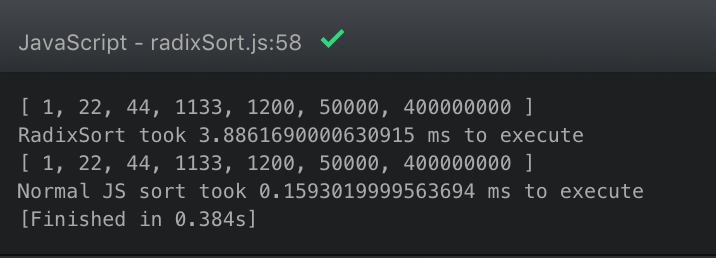## Why Care?

A friend of mine was interested in an idea for beating the casino at roulette.

His theory is that if he keeps playing either red or black, but adding to the bet, there is a mathematical certainty of winning at some point.

First spin, go red with \$10.

Second spin, go red with \$22.

Third spin, go red with \$33.

Or something like that.

I told him I could write a program that would tell him the number of consecutive results from 50/50 roulette spins.

This is how we did it:

## Tutorial For Launching Simple Algorithm Tools

### Learning Algorithmic Technology

At this tutorial’s core, this is an HTML5 tutorial.

HTML5 incorporates HTML and JavaScript together. HTML5 incorporates CSS as well, but this tutorial doesn’t discuss that because design hasn’t been addressed here.

This tutorial takes the watcher on a journey of creating a simple web tool. In it we:

• Start a project using command line interface (CLI) tools
• Write the JavaScript algorithmic logic for the probability
• Write the HTML5 user input form and clickable submit button
• Link the JavaScript algorithm and the HTML5 input to work as a graphic user interface
• Write clear directions for what the tool is used for
• Initialize the project as a git project (version history software)
• Sync our project with a GitHub repository
• Publish the tool using GitHub pages

The tool is now available on the internet to whomever cares to view it. Click here to check it out:

www.ianrobinson.net/roulettespinner

If I were to answer my buddies specific question, this tool need more work. We would need to incorporate the increments of betting in order for us to get a specific plan to beat roulette…. but at the end of the day, this isn’t about beating the casinos. Even if we started beating casinos, the casinos would just kick us out.

The cool thing about this is that it helps us think algorithmically about complex issues. Using computers as tools, we can run massive tests in the blink of an eye. My computer only starts to have slow results (requiring 2-3 seconds) around 100 million spins. Imagine how long it would take to do 100 million spins in real life.

Algorithms are fun.

## Radix Sort is Fun… but it’s Useless in JavaScript

I just spent an hour writing a Radix sort algorithm. It turns out that it is 25% the speed of the built in JavaScript `.sort()` function.Apparently all this sorting has been figured out in the past. Strange that it’s part of learning to code these days…

```const performance = require('perf_hooks').performance;

function getDigit(num, location) {
let stringNum = num.toString();
let resultNum = parseInt(stringNum[stringNum.length - 1 - location]);
if (resultNum) {
return resultNum;
} else {
return 0;
}
}

function getDigitCount(num) {
let digitCount =  Math.abs(num).toString().length;
return digitCount;
}

function mostDigits(arr) {
let maxDigits = -Infinity;
for (var i = 0; i < arr.length; i += 1) {
var digitCount = getDigitCount(arr[i])
if (maxDigits < digitCount) {
maxDigits = digitCount;
}
}
return maxDigits;
}

let numberOfDigitsTheLargestNumberHas = mostDigits(list);

for (let i = 0; i < numberOfDigitsTheLargestNumberHas; i += 1) {
let bucket = [[],[],[],[],[],[],[],[],[]];

for (var j = 0; j < list.length; j += 1) {
let number = list[j];
bucket[getDigit(number, i)].push(number);
}
list = bucket.flat(2);
}
return list.flat();
}

function sum(a, b) {
return a - b;
}

var ourBeautifulArray = [50000, 1200,1133, 44, 22, 1, 400000000];
var time1 = performance.now();
var time2 = performance.now();
console.log(`RadixSort took \${time2 - time1} to execute`);

var time3 = performance.now();
console.log(ourBeautifulArray.sort(sum));
var time4 = performance.now();
console.log(`Normal JS sort took \${time4 - time3} to execute`);
```

It’s possible that there is room for improvement in the way I’m executing this algorithm. Perhaps using `.flat` is causing this.

Either way, even if it’s not a useful thing to be able to do, I think it’s interesting. Once you figure out how it works, it’s neat because it sorts things in non-obvious ways.

I just wish it performed better. LoL

If you want to see the code I’m working with to deepen my knowledge of JavaScript, check out this repository.

This is in response to a FreeCodeCamp curriculum session which teaches spread operators.

I found FCC’s explanation of spread operators (click here) to be a very complex description of a simple concept.

Thank you to Brandon Morrelli’s article which provided a clear description of how spread operators can be used to make it easy to work with arrays.

## How the Spread Operator Works

Here is the simple way of understanding how a spread operator works:

```var arr1 = ['three', 'four'];

var arr2 = ['one', 'two', ...arr1, 'five', 'six'];

console.log(arr2);
// logs [ 'one', 'two', 'three', 'four', 'five', 'six' ]```

Essentially the spread operator allows us to inject arrays into spots where we want only the elements, not the array container.

Here is an example of what happens when we don’t have a spread operator:

```var arr1 = ['three', 'four'];

var arr2 = ['one', 'two', arr1, 'five', 'six'];

console.log(arr2);
// logs [ 'one', 'two', [ 'three', 'four' ], 'five', 'six' ]```

In the example above, we have a nested array which could be problematic depending on the architecture of your application.

Essentially, this makes it so we can evaluate arrays in place.

## ES6: Use the Spread Operator to Evaluate Arrays In-Place

This is the answer that passes the tests in the current version of Free Code Camp:

```const arr1 = ['JAN', 'FEB', 'MAR', 'APR', 'MAY'];
let arr2;
(function() {
"use strict";
arr2 = [...arr1];
})();
console.log(arr2);```